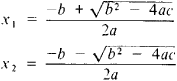Also found in: Dictionary, Thesaurus, Acronyms, Wikipedia.

(mathematics)
Any second-degree polynomial equation.

an equation of the form ax2 + bx + c = 0, where a, b, and c are any number and are called the coefficients of the equation. A quadratic equation has two roots, which are found by the formulasThe expression D = b2 − 4ac is called the discriminant of the quadratic equation. If D > 0, then the roots of the quadratic equation are real and unequal; if D < 0, then the roots areconjugate complex numbers; if D = 0, then the roots are real andequal. The Vièta formulas x1 + x2 = − b/a and x\x2 = c/a link the roots and coefficients of a quadratic equation. The left-hand side of a quadratic equation can be expressed in the form α (xx2) (xx2). The function y = ax2 + bx + c is called a quadratic trinomial, and its graph is a parabola with the vertex at the point M (−b/2a; cb2/4a) and axis of symmetry parallel to the. y-axis; the direction of the branches of the parabola coincides with the sign of a. The solution of the quadratic equation was already known in geometric form to ancient mathematicians.

References in periodicals archive ?
Kids who look up the quadratic equation may end up like the child who looked up "meticulous"; they have a definition, but they don't have the background knowledge to use it correctly.
AA, "Solution of Algebraic Quadratic Equations Taking into Account Transitional Processes in Oscillation Systems", General Mathematical Notes 2010a, 1.
Various algebraic identities and constructions equivalent to the solution of quadratic equations were expressed and proven in geometric form.
2] = -1/[phi] are the solutions to the quadratic equation [a.
As the first author's work with the teachers indicates, such understanding results from one's conceptualization of the locus as an equivalent representation of the quadratic formula, including geometric interpretation of what it means for a discriminant of a quadratic equation to be a factorable or nonfactorable algebraic expression.
As an extreme example, suppose a class of 25 ninth-graders were exposed to one minute of instruction on how to solve quadratic equations.
When the OR-TL relation was exponentially shaped and all annuli were used, the least error resulted from direct substitution into the fitted quadratic equation (Fig.
up] were the two roots of the piecewise quadratic equation
With these verbal analogues of the quadratic equation we already have more than enough for our minds to ruminate upon for quite some time.
To determine the impact of temperature on the detectors, Parola used the software to develop a quadratic equation.
MATHEMATICAL EXPRESSION NOT REPRODUCIBLE IN ASCII] The reduced stress term is expressed in the form of a quadratic equation in ([I.
A quadratic equation was fitted to the data and the index was scaled to 100% at 1934 (Fig.

Site: Follow: Share:
Open / Close